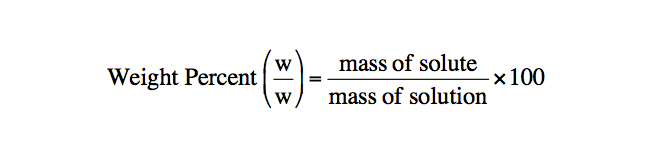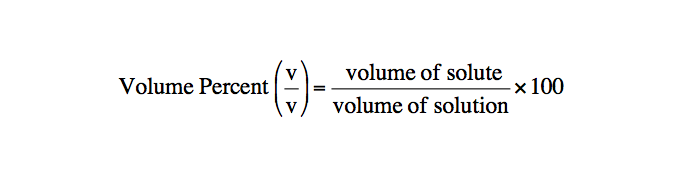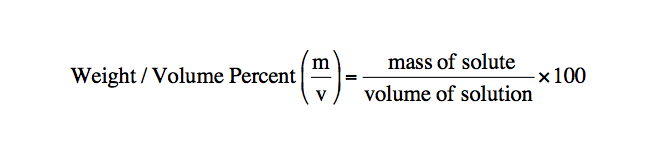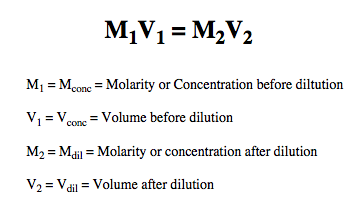Clutch Prep is now a part of Pearson
Ch.1 - Chemical MeasurementsWorksheetSee all chapters

# Volumetric Analysis

See all sections
Sections
SI Units
Metric Prefixes
Chemical Concentrations
Volumetric Analysis
Volumetric Titrations

The concentration of solutions can be expressed in terms beyond molarity and molality.

###### Percent Composition

Concept #1: The concentration of solutions can further be discussed when reviewing weight percentvolume percent, and weight/volume percent

Weight percent represents the mass composition of solute within a solution.Volume Percent represents the volume composition of solute within a solution.Weight/Volume Percent combines aspects of both weight percent and volume percent.Example #1: A solution is prepared by dissolving 18.83 g sulfuric acid, H2SO4, in enough water to make 250 ml of solution. If the density of the solution is 1.1094 g/mL, what is the weight % H2SO4 in the solution? (MW of H2SO4 is 98.086 g/mol).

Example #2: When lead levels in blood exceed 0.80 ppm (parts per million) the level is considered dangerous. 0.80 ppm means that 1 million g of blood would contain 0.80 g of Pb. Given that the density of blood is 1060.0 kg/m3, how many grams of Pb would be found in 550.00 mL of blood with a Pb level of 0.583 ppm?

###### Percent Composition Calculations

Example #3: A 8.13% Al2(SO4)3 solution ( MW of Al2(SO4)3 is 342.17 g/mol ) has a measured density of 1.235 g/mL. Calculate the molar concentration of sulfate ions in the solution.

Example #4: The density of a 33.8% solution of sodium acetate, NaC2H3O2, is 1.10 g/mL. A reaction requires 68.8 g NaC2H3O2. What volume of the solution do you need if you want to use a 50% excess of NaC2H3O2? (MW of NaC2H3O2 is 82.034 g/mol).

Practice: How many grams of nitric acid, HNO­3, and water are found in 53.1 g of 83.1 mass percent nitric acid?

###### Dilutions

Example #5: A dilution involves the addition of water to a solution in order to make it less concentrated.Example #6: How many grams of 53.1 weight % NaCl (MW of NaCl is 58.443 g/mol) should be diluted to 2.50 L to make 0.15 M NaCl?

Practice: If 920 mL of water is added to 78.0 mL of a 1.28 M HBrO4 solution what is the resulting molarity?

###### Dilutions Calculations

Example #7: Calculate the density of 15.9 mL of a 49.1% by weight of aqueous perchloric acid, HClO4, when it is diluted to 825 mL of 0.100 M HClO4? (MW of HClO4 is 100.461 g/mol)

Example #8: The density of 63.7 wt% NaOH is 0.915 g/mL. How many milliters of water should be diluted to 850.0 mL to create 0.425 M NaOH?